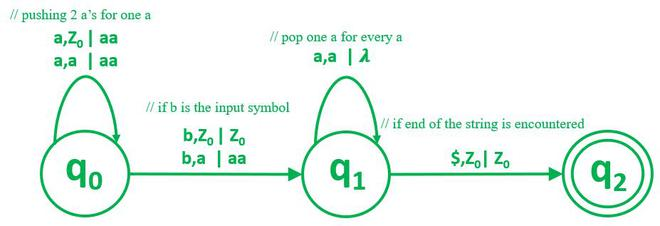Open in App
Not now

# Construct Pushdown automata for L = {a^n b a^2n | n ≥ 0}

• Difficulty Level : Hard
• Last Updated : 11 Nov, 2021

Prerequisites:

Problem– Construct PDA for the language L = {anba2n | n ≥ 0}

This means the PDA should have twice as many as a’s after b than before b, and there should be one and only one b.

Examples:

```INPUT : aaabaaaaaa
OUTPUT : Accepted```
```INPUT : aaaaabaaaa
OUTPUT : Rejected```
```INPUT : NULL STRING
OUTPUT : Rejected ```

Approach:

Push twice as many a’s for every a that we receive as an input symbol. We keep repeating this till we receive b. Once, the input symbol is b, skip b and pop one a for every a.

NOTE: For every such question when the number of a certain symbol is a multiple of another symbol, the key is to make the count of both the symbols, equal.

```1. <STATE q0 > At empty stack if we get 'a' as input symbol, push 2 a's to the stack.
2. If we get 'b' as input symbol we move to q1.
3. <STATE q1 > For every a that we get as input symbol now, we pop 1 a.
4. If end of string symbol is encountered then transit to q2.```

The required Pushdown Automata:My Personal Notes arrow_drop_up